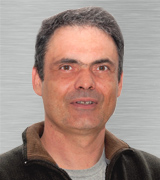Past Event: Babuška Forum

# Verification and Validation in Computational Sciences and Engineering

Serge Prudhomme, Professor, Mathematical and Industrial Engineering, Polytechnique Montréal

10 – 11AM
Friday Sep 22, 2023

POB 6.304 & Zoom

## Abstract

Verification and Validation are essential activities for predictive modeling in computational sciences and engineering. In this presentation, I will focus on two topics, namely the verification of neural network approximations of solutions to boundary-value problems and the optimal design of experiments for validation of mathematical models.

In the first part of the talk, I will present the multi-level neural network approach capable of reducing the numerical error to machine precision. The solution to partial differential equations using deep learning approaches has shown in recent years promising results. However, the ability of these methods to surpass classical discretization methods remains a significant challenge. One of their main obstacles lies in their inability to consistently reduce the relative error in the computed solution. To better control the error, the proposed approach consists in estimating the correction in a previously computed neural network approximation by solving the problem for the residual error using a new network of increasing complexity.

In the second part of the talk, I will present a novel methodology to design an optimal validation scenario to assess the predictivity of a model in the cases where experimental data at the prediction scenario are unavailable and the quantity of interest cannot be observed. To do so, we propose to solve two optimal design problems that best match the sensitivity indices that one would obtain at the prediction scenario. In particular, the first optimization problem identifies an optimal validation scenario such that the sensitivities in the quantity of interest at the validation and prediction scenarios are alike. The second optimal design problem allows one to identify the most relevant observations to be performed. The methodology will be illustrated on two examples. The first example, dealing with a free-falling object, demonstrates the importance of the choice of the validation experiment. The second example, involving a pollutant transport problem, shows how the validation scenario should depend on the choice of the quantity of interest.

## Biography

Serge Prudhomme is a Professor of Mathematical and Industrial Engineering at Polytechnique Montréal. He received his Ph.D. in Aerospace Engineering and Engineering Mechanics from UT Austin within the Texas Institute for Computational and Applied Mathematics (TICAM). His research interests include a posteriori estimation, adaptive methods, uncertainty quantification, and multiscale and reduced-order modeling.### Event information

Date
10 – 11AM
Friday Sep 22, 2023
Location POB 6.304 & Zoom
Hosted by Blake Christierson